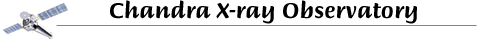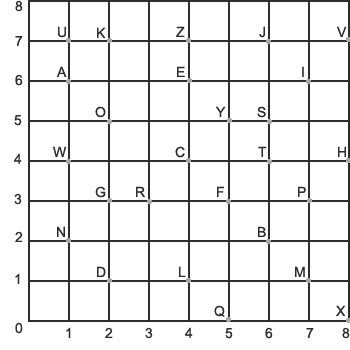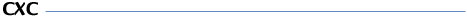## Break the Code!Answer the questions, using the locations in the grid above to put them in code.
Example: Science = (6,5) (4,4) (7,6) (4,6) (1,2) (4,4) (4,6)

1. What is your name in code?
2. This X-ray observatory is named after a famous astrophysicist.
Chandra: (4,4) (8,4) (1,6) (1,2) (2,1) (3,3) (1,6)
3. We call ours the Milky Way.
Galaxy: (2,3) (1,6) (4,1) (1,6) (8,0) (5,5)
4. This was the 1st Space Shuttle commanded by a woman, mission STS-93.
Columbia: (4,4) (2,5) (4,1) (1,7) (7,1) (6,2) (7,6) (1,6)
5. The study of the stars.
Astronomy: (1,6) (6,5) (6,4) (3,3) (2,5) (1,2) (2,5) (7,1) (5,5)
6. Chandra detects these to observe space.
X-rays: (8,0) - (3,3) (1,6) (5,5) (6,5)
7. When a star explodes it is called this.
Supernova: (6,5) (1,7) (7,3) (4,6) (3,3) (1,2) (2,5) (8,7) (1,6)
8. Nothing can escape the pull of this object, not even light.
Black Hole: (6,2) (4,1) (1,6) (4,4) (2,7) _ (8,4) (2,5) (4,1) (4,6)
9. The US government organization that directs space missions.
NASA: (1,2) (1,6) (6,5) (1,6)
10. A ball of gas, such as our Sun.
Star: (6,5) (6,4) (1,6) (3,3)